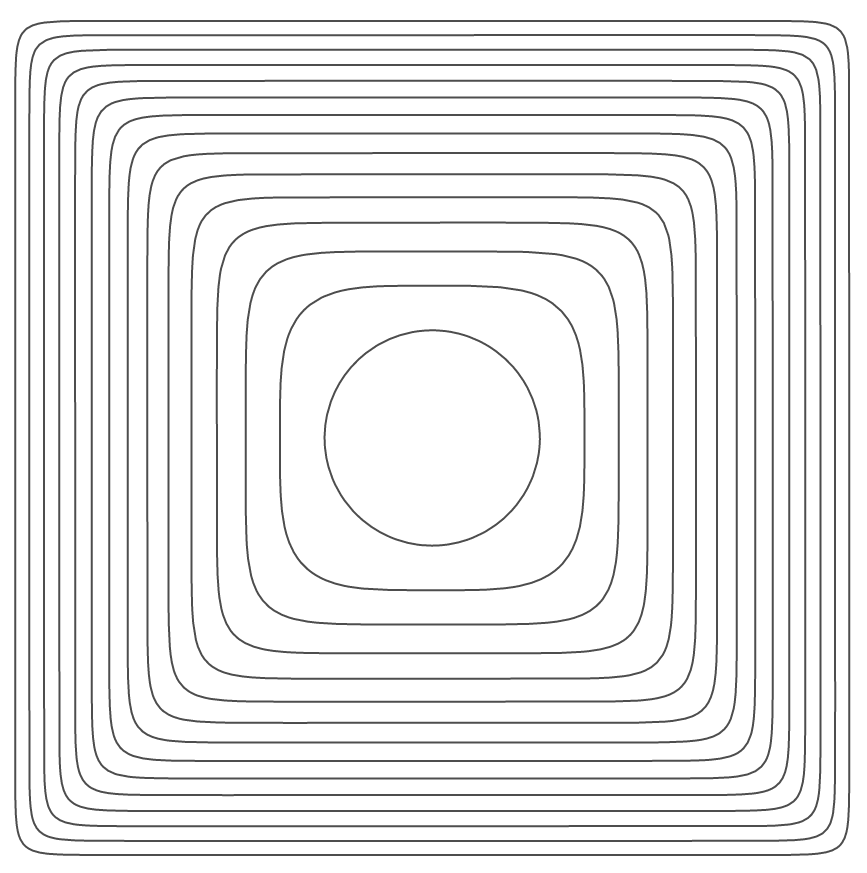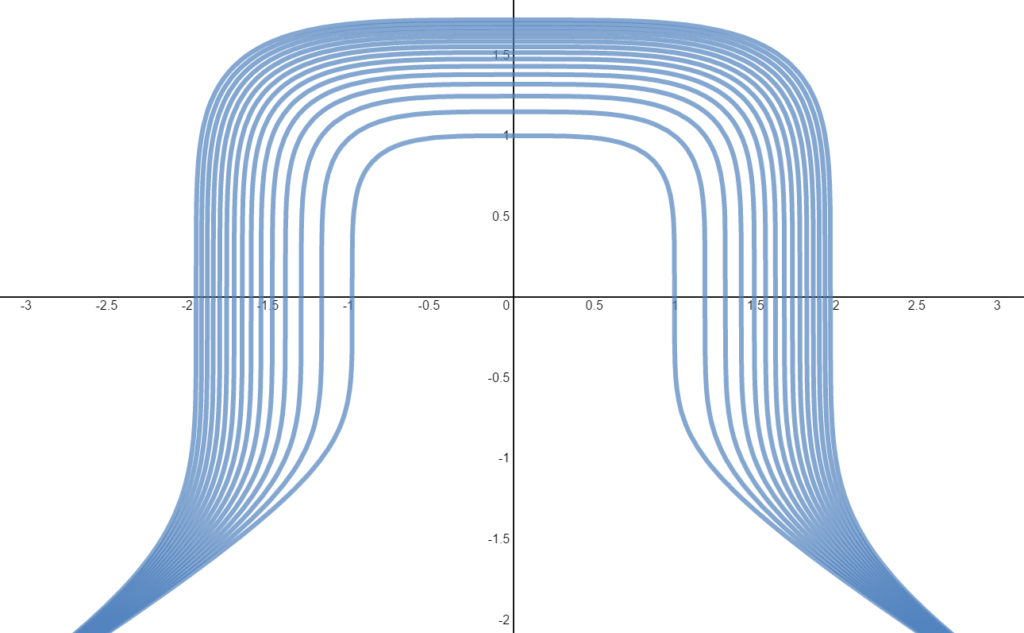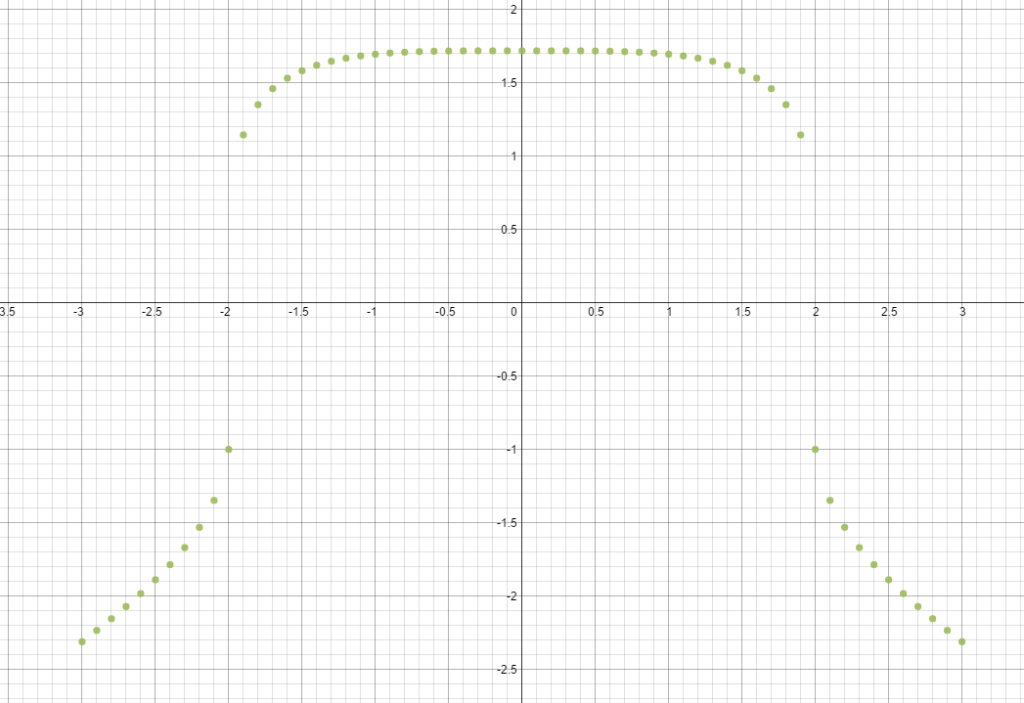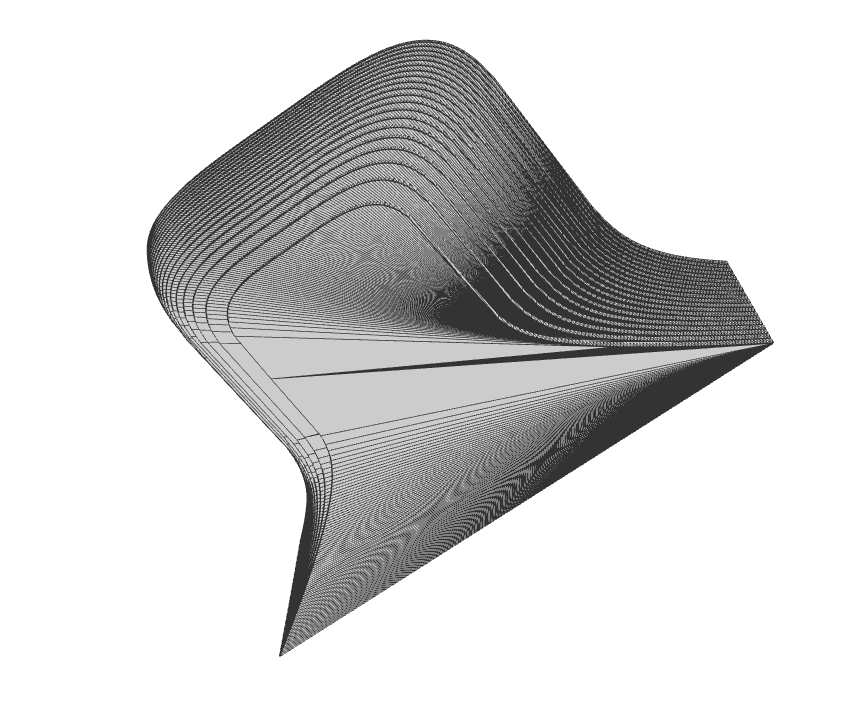# Workflow: Daily Desmos -> Desmos -> Madeup -> 3D Print

The seniors don’t go to school on the last day, so I had calculus class on Friday with one student (a junior). We went down to the innovations lab to create things. Here’s what we worked on:I had the student work on the solution after talking about superellipses (or the better name, squircles).

When he finished the solution, we modified it to get this sketch:and we decided to try and get a 3D print of this sketch. We started working in madeup (a fantastic beta of a program that takes code and creates 3D models), but found it tricky to graph the function. Went back to desmos to get this sketch where we could figure out how to get the specific coordinates of points along the curve.Fiddled around for a bit to get this result:Here’s the code:

```to abs x = if x > 0 then x else -x

to func t_ a_
if (t_ < 0)
t_ = t_ * -1
end
out = a_ - t_ ^ 4
if (out < 0)
out = abs out
out = out ^ (0.2)
out = - out
else
out = out ^ (0.2)
end
out
end

a = 20
h = 0
while a > 0
t = -3
while t < 3
x = t
y = func t,a
moveto x,y,h
t = t + 0.025
end
h = h + 0.1
extrude 0,0,1,-0.1
a = a - 1
end
```